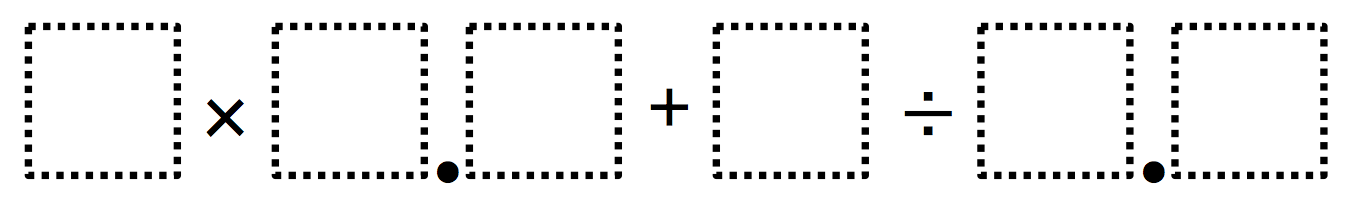# Evaluating a Decimal Expression

Directions: Using the digits 0 to 9 at most one time each, fill in the boxes to make the value of this expression is as large as possible. Challenge: Try to make this expression as close to 30 as possible (for an added challenge, try to make this expression as close to 30 as possible using just the digits 1 through 9).### Hint

How can we figure out which part of the expression has the biggest impact on the answer? The smallest impact?

The largest (using 0-9) value is 8 x 7.6 + 9/0.1 = 150.8
You can make exactly 30 several ways (using 0-9) including:
4 x 7.5 + 0/1.2 = 30
7 x 4.0 + 3/1.5 = 30
6 x 4.5 + 9/3.0 = 30
If you only use digits 1-9 this is harder but you can still get exactly 30: 3 x 7.5 + 9/1.2 = 30

Source: Erick Lee

## Add Fractions with Decimal Sums

Directions: Using the digits 1 to 9 at most one time each, place a digit …

1.2.